# Random Maths Lesson Starters

If you are looking for a Maths Lesson Starter that can be used more than once with the same class this is the place for you.

The starters here have been selected because the numbers will change every time the page is loaded. Students could practise the techniques week after week knowing that they are dealing with different figures each time.

Main Page

### Random Starters: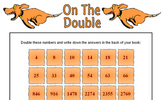On The Double: Double the numbers given in the table. An optional student activity is linked from the bottom of this starter page called Doubling Quiz. Drag the number cards onto their doubles. No calculators or writing allowed..Add Quickulations: Calculations appear on the screen every few seconds. This mental arithmetic starter provides pace to the start of the Maths lesson. An optional student activity is linked from the bottom of this starter page called Basic Addition. A self-marking exercise on addition with increasing levels of difficulty..Six of the Best: Six calculations to perform without a calculator. An optional student activity is linked from the bottom of this starter page called Number Skills Inventory. A checklist of basic numeracy techniques that every pupil should know..Middle of Centres: The blue point is exactly in the middle of two red points. What are their coordinates? An optional student activity is linked from the bottom of this starter page called Where's Wallaby?. Find the hidden wallaby using the clues revealed at the chosen coordinates..Outnumbered: Which group of four numbers, arranged in a square, has the largest total? An optional student activity is linked from the bottom of this starter page called Discombobulated. A fun game requiring you to find numbers which add up to the target number as quickly as possible..Guitar For Sale: A question about the profit made when buying and selling a guitar. An optional student activity is linked from the bottom of this starter page called Aunt Lucy's Legacy. Decide which of the four schemes Aunt Lucy proposes will provide the most money. This investigation involves the sum of sequences as well as considering life expectancy..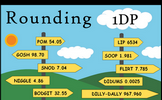Rounding 1dp: Round off the given numbers to 1 decimal place then add the answers together. An optional student activity is linked from the bottom of this starter page called Rounding DP. A self marking exercise requiring students to round numbers to a given number of decimal places..Pie Chart: An exercise in estimating what the sectors of a pie chart represent. An optional student activity is linked from the bottom of this starter page called Pie Charts. Develop the skills to construct and interpret pie charts in this self-marking set of exercises..Memor Sea: Twelve numbers in a seascape need to be memorised in order to answer the five questions. An optional student activity is linked from the bottom of this starter page called Screen Test. Memorise the mathematical facts in the video then answer the ten quiz questions..Word Search: Create your own mathematical word search for the whole class to enjoy. An optional student activity is linked from the bottom of this starter page called Word Search. Find the mathematical words in the grid of letters..Arithmagons: This lesson starter requires pupils to find the missing numbers in this partly completed arithmagon puzzle. An optional student activity is linked from the bottom of this starter page called Arithmagons. An extensive collection of Arithmagons arranged in levels according to their features. Find the missing numbers in these online, self-checking exercises and discover the wonders of Arithmagons..What are they?: A starter about sums, products, differences, ratios, square and prime numbers. An optional student activity is linked from the bottom of this starter page called What Are They?. An online exercise about sums, products, differences, ratios, square and prime numbers..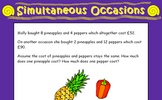Simultaneous Occasions: A problem which can best be solved as a pair of simultaneous equations. An optional student activity is linked from the bottom of this starter page called Simultaneous Equations. A self-marking, multi-level set of exercises on solving pairs of simultaneous equations..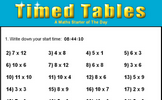Timed Tables: How fast can you answer 24 mixed times tables questions? An optional student activity is linked from the bottom of this starter page called Times Tables. A collection of activities to help you learn your times tables in only 5 days..Coloured Sheep: What is the probability of picking a red sheep from the sheep in the field? An optional student activity is linked from the bottom of this starter page called Likelihood. Arrange some statements in order according to the probability of them happening. Compare your opinion with thousands of others..Writing Cheques: Complete some imaginary cheques, the amount needs to be written in words. An optional student activity is linked from the bottom of this starter page called Numbers in Words. Find the five lettered mathematical words by matching numbers with their equivalent in words..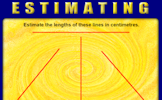Estimating: Estimate the lengths of four lines then add your answers together. An optional student activity is linked from the bottom of this starter page called Rough Answers. An exercise on rounding values in a calculation to find an approximate estimate of the answer..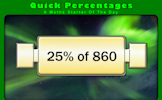Quick Percentages: Simple percentage questions appear on screen then fade every 8 seconds. This Starter is customisable. An optional student activity is linked from the bottom of this starter page called Express as a Percentage. This self-marking quiz requires you to work out what one quantity is as a percentage of a second quantity..Numbers in words: Write out in words some numbers writen as digits (optional pirate theme) An optional student activity is linked from the bottom of this starter page called Words in Digits. Write the numbers given in words as digits and vice versa..No Partner: Find which numbers in a given list do not combine with other numbers on the list to make a given sum. An optional student activity is linked from the bottom of this starter page called No Partner. Find which numbers in a given list do not combine with other numbers on the list to make a given sum..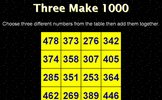Three Make 1000: Find three numbers from those given that add up to 1000. How many different sets of three numbers can be found? An optional student activity is linked from the bottom of this starter page called . .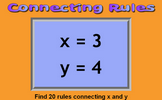Connecting Rules: Give 20 rules connecting x and y given their values. An optional student activity is linked from the bottom of this starter page called . .Structured Settlement: Without a calculator match a a pie slice to a calculation to an answer. An optional student activity is linked from the bottom of this starter page called Pie Charts. Develop the skills to construct and interpret pie charts in this self-marking set of exercises..Clouds: Some calculations are partly obscured. Work out what those calculations might be. An optional student activity is linked from the bottom of this starter page called Clouds. Can you work out which numbers are hidden behind the clouds in these calculations?.Product Practice: Work out the answers to the given calculations then add the answers together. There are three different levels of difficulty. An optional student activity is linked from the bottom of this starter page called Ten Multiplications. Twelve multiplications for you to do without a calculator..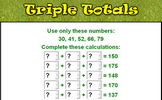Triple Totals: Complete the sums using only the given numbers then check your calculations are correct. An optional student activity is linked from the bottom of this starter page called Mixpressions. Arrange the cards to create a valid mathematical statement..Tasty Take-Aways: Four subtractions to be done without a calculator. The answers are then to be added together. An optional student activity is linked from the bottom of this starter page called Ten Subtractions. Ten subtractions for you to do without a calculator..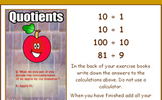Quotients: Work out the answers to the given division calculations then add the answers together. An optional student activity is linked from the bottom of this starter page called Twelve Divisions. Twelve divisions for you to do without a calculator..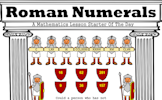Roman Numerals: Learn a very different method for writing numbers using Roman numerals. An optional student activity is linked from the bottom of this starter page called Roman Numerals Jigsaw. An online interactive jigsaw puzzle of a grid of Roman numerals..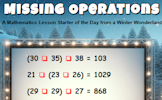Missing Operations: Each red box represents a missing operation (+, -, x or ÷). Can you work out what they are? An optional student activity is linked from the bottom of this starter page called BIDMAS. A self marking exercise testing the application of BIDMAS, an acronym describing the order of operations used when evaluating expressions..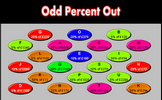Odd Percent Out: A number of simple percentage calculations are given. The answers are in pairs. Which is the odd one out? An optional student activity is linked from the bottom of this starter page called Percentages. A multi-level quiz on finding percentages. The lower level questions can be done mentally while the highest level questions require a calculator..Figuratively Speaking: Write the numbers written as words using digits. An optional student activity is linked from the bottom of this starter page called Words in Digits. Write the numbers given in words as digits and vice versa..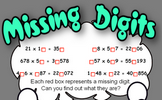Missing digits: Work out what the missing digits in the calculations are. An optional student activity is linked from the bottom of this starter page called Maths Advent Calendar. One window to open for each of the first 25 days of December leading to mathematical puzzles, games or activities..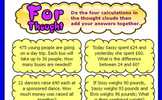For Thought: Add up the answers to the four real life questions. An optional student activity is linked from the bottom of this starter page called How Many of Each?. Work out how many items were bought from the information given..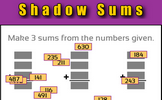Shadow Sums: Make sums from the three digit numbers given. An optional student activity is linked from the bottom of this starter page called Twelve Sums. A self-marking exercise on addition with increasing levels of difficulty..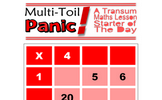Multi-Toil Panic: Copy and complete the multiplication grid. The higher levels include negative numbers. An optional student activity is linked from the bottom of this starter page called Clouds. Can you work out which numbers are hidden behind the clouds in these calculations?.

### Other

Is there anything you would have a regular use for that we don't feature here? Please let us know.

Contact Transum

#### Perfect Magic SquareArrange the sixteen numbers on the four by four grid so that groups of four numbers in a pattern add up to the same total. So far this activity has been accessed 149 times and 3 people have earned a Transum Trophy for completing it.

### Feedback

Do you have any comments? It is always useful to receive feedback and helps make this free resource even more useful for those learning Mathematics anywhere in the world. Click here to enter your comments.For All: